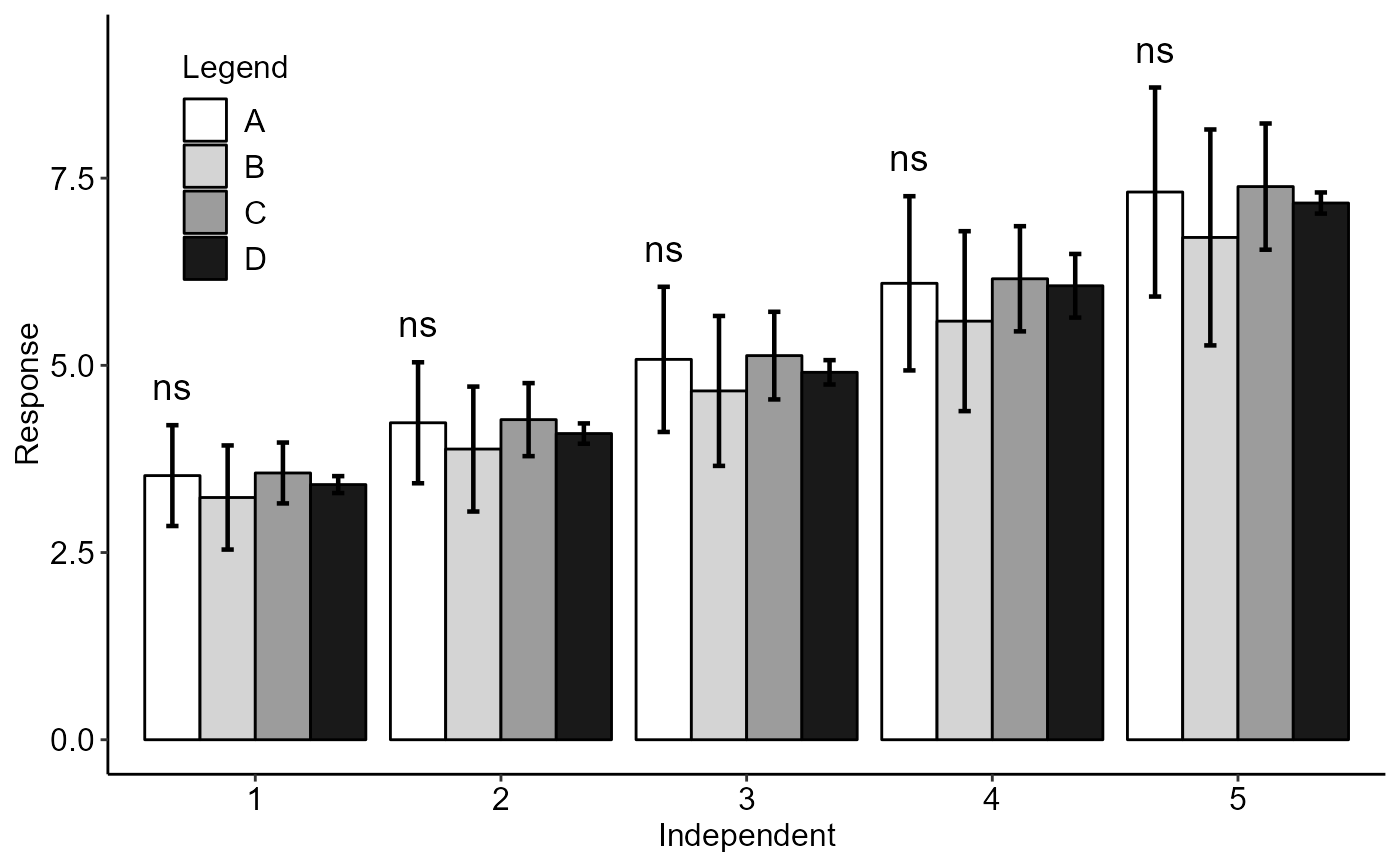Function of the AgroR package for the analysis of experiments conducted in a balanced qualitative single-square Latin design with multiple assessments over time, however without considering time as a factor.

DQLT(
trat,
line,
column,
time,
response,
alpha.f = 0.05,
alpha.t = 0.05,
mcomp = "tukey",
error = TRUE,
xlab = "Independent",
ylab = "Response",
textsize = 12,
labelsize = 5,
pointsize = 4.5,
family = "sans",
sup = 0,
posi = c(0.1, 0.8),
geom = "bar",
fill = "gray",
legend = "Legend",
ylim = NA,
width.bar = 0.2,
size.bar = 0.8,
dec = 3,
theme = theme_classic(),
xnumeric = FALSE,
all.letters = FALSE
)

## Arguments

trat

Numerical or complex vector with treatments

line

Numerical or complex vector with line

column

Numerical or complex vector with column

time

Numerical or complex vector with times

response

Numerical vector containing the response of the experiment.

alpha.f

Level of significance of the F test (default is 0.05)

alpha.t

Significance level of the multiple comparison test (default is 0.05)

mcomp

Multiple comparison test (Tukey (default), LSD, Scott-Knott and Duncan)

error

xlab

Treatments name (Accepts the expression() function)

ylab

Variable response name (Accepts the expression() function)

textsize

Font size of the texts and titles of the axes

labelsize

Font size of the labels

pointsize

Point size

family

Font family

sup

Number of units above the standard deviation or average bar on the graph

Plot the average value on the graph (default is TRUE)

posi

Legend position

geom

Graph type (columns - "bar" or segments "point")

fill

Defines chart color (to generate different colors for different treatments, define fill = "trat")

legend

Legend title

ylim

Define a numerical sequence referring to the y scale. You can use a vector or the seq command.

width.bar

width error bar

size.bar

size error bar

dec

Number of cells

theme

ggplot2 theme (default is theme_classic())

xnumeric

Declare x as numeric (default is FALSE)

all.letters

Adds all label letters regardless of whether it is significant or not.

## Value

The function returns the p-value of Anova, the assumptions of normality of errors, homogeneity of variances and independence of errors, multiple comparison test, as well as a line graph

## Details

The p-value of the analysis of variance, the normality test for Shapiro-Wilk errors, the Bartlett homogeneity test of variances, the independence of Durbin-Watson errors and the multiple comparison test ( Tukey, Scott-Knott, LSD or Duncan).

## Note

The ordering of the graph is according to the sequence in which the factor levels are arranged in the data sheet. The bars of the column and segment graphs are standard deviation.

## Author

Gabriel Danilo Shimizu, shimizu@uel.br

Leandro Simoes Azeredo Goncalves

Rodrigo Yudi Palhaci Marubayashi

## Examples

rm(list=ls())
data(simulate3)
attach(simulate3)
#> The following objects are masked from simulate1:
#>
#>     resp, tempo, trat
#> The following objects are masked from aristolochia (pos = 4):
#>
#>     resp, trat
#> The following objects are masked from simulate2:
#>
#>     resp, tempo, trat
#> The following objects are masked from laranja:
#>
#>     resp, trat
#> The following objects are masked from aristolochia (pos = 7):
#>
#>     resp, trat
#> The following object is masked from cloro:
#>
#>     resp
#> The following object is masked from passiflora:
#>
#>     trat
DQLT(trat, linhas, colunas, tempo, resp)
#>
#> -----------------------------------------------------------------
#> ANOVA and assumptions
#> -----------------------------------------------------------------
#>   p-value ANOVA Shapiro-Wilk  Bartlett Durbin-Watson   CV (%)
#> 1     0.8315413    0.1585848 0.7511662     0.2932159 15.99516
#> 2     0.8315413    0.1585848 0.7511662     0.2932159 15.99516
#> 3     0.8315413    0.1585848 0.7511662     0.2932159 15.99516
#> 4     0.8211590    0.1062631 0.8433703     0.2928187 15.81922
#> 5     0.8656823    0.1087673 0.7100666     0.2383646 17.44844